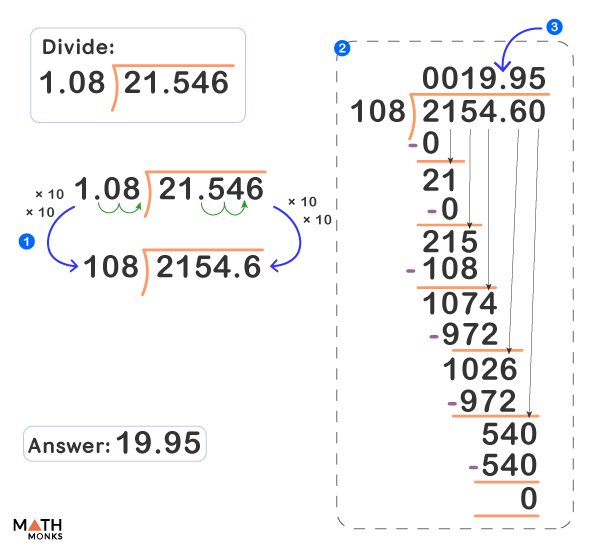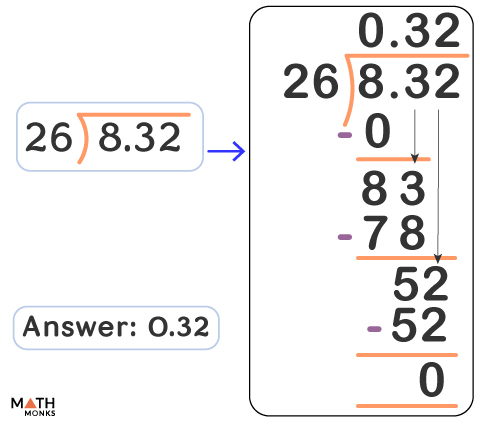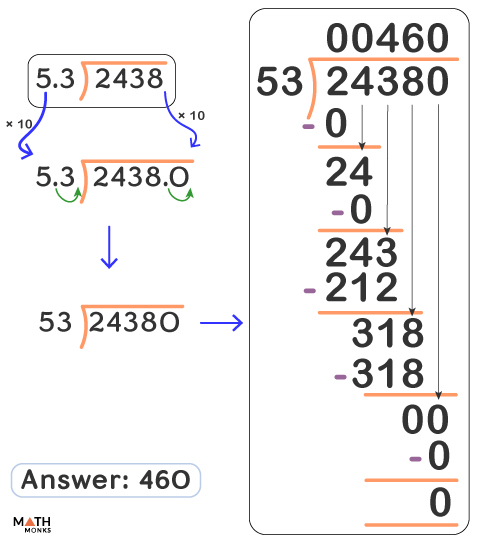# How to Divide Decimals

Dividing with decimals is very useful whether we calculate the dinner bill, fill up our car fuel tank, or split the recipe ingredients. We need to follow some simple steps to divide decimals.

Let us try a simple division using models shown in the example below.

But we cannot use models while dividing decimals. So we will learn the rules (steps) to divide decimals.

## Rules on Dividing Decimals

Let us first learn how to divide decimals by decimals.

1. Move the decimal point in the divisor to the right until it becomes a whole number.
2. Move the decimal point to the places as much as in the divisor.
3. Now divide.

Let us divide 4.48 by 1.6.

Divide:  4.446 ÷ 0.19Solution:

The solution is given in the diagram alongside.
We move the decimal point 2 places to the right in 0.19 and change it to 19.
We do the same in 4.446 and change it to 444.6.
∴ 444.6 ÷ 19 = 23.4

Dividing decimals in LONG DIVISION.

Divide: 21.546 ÷ 1.08.Solution:

The solution is given in the diagram alongside.

## Dividing Decimals with Whole Numbers

### A Decimal by a Whole Number

We see another simple example below where we use the standard rule of dividing decimals. Here we do not have to convert the divisor to a whole number. We divide and put the decimal point accordingly.

Divide:  8.32 ÷ 26.Solution:

The solution is given in the diagram alongside.

### A Whole Number with a Decimal

We apply the standard rule when dividing a whole number by a decimal. We multiply both the dividend and the divisor with 10. Then we move the decimal point to the right so that the divisor becomes a whole number. In such divisions, the dividend gets larger by 10x or 20x or so on.

An example is shown below: